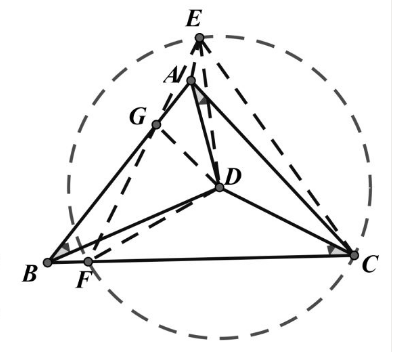【答案】

$\triangle A B C$ 是正三角形。

$D A 、 D B 、 D C$ 互不相等, 不妨设 $D A$ 最小,
$D B$ 最大。以 $D$ 为圆心, $D C$ 为半径作圆,

$\angle D A C=\angle D E C=30^{\circ}$, 有 $D 、 A 、 E 、 C$

$\angle A E D=\angle A C D < 30^{\circ}$, 有点 $A$ 在 $\triangle F E D$ 内部。

$\therefore \angle F G D=\angle F B D < \angle C F D=30^{\circ}$, 而 $\angle F G D > \angle F E D=30^{\circ}$ 这是矛盾的。